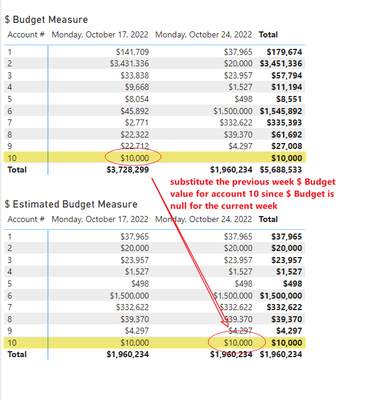cancel
Showing results for
Did you mean:

Fabric is Generally Available. Browse Fabric Presentations. Work towards your Fabric certification with the Cloud Skills Challenge.New Member

## Finding Total Sum of an "if then" statement

I'm trying to find the total sum of an "IF/Then" Statement. The budget is set forward by individuals in our company but some of them aren't diligent with updating the budget of the current week. So I set forward an if/then statement to try and capture the budgets they input in the previous week. But when it comes to summing the total it only sums up the current week budgets and ignores the previous week. Is there a way for me to find the total sum of the current budget, and the previous week (if they forgot to update the budget to the current week)?

Here's my current DAX and a sample of how the data looks. Currently the Budget data is in a separate table from my date table hence why I have to use a filter in order to connect them.

This Month Expected =

var WeekStart = today() - WEEKDAY(today(),2)

var WeekEnd = today()- WEEKDAY(today(),2) + 6

var pastweek = today() - WEEKDAY(today(),2) - 6

var pastweekend = today() - WEEKDAY(today(),2) - 2

return
calculate(if(isblank(calculate(sum([budget]), filter(all('date table'), [Date] >= WeekStart && [Date] <= WeekEnd))), calculate(sum([budget]), filter(all('date table'), 'date table'[Date] >= pastweek && 'date table'[Date] <= pastweekend)), calculate(sum([budget]), filter(all('date table'), 'date table'[Date] >= WeekStart && 'date table'[Date] <= WeekEnd))))

 pk_date Date 1 1/1/2021 2 1/2/2021

 Budget fk_date Account 1000 1 1 3000 1 2 2000 1 3 3500 2 1 4000 2 2
6 REPLIES 6New Member

Here's a sample PBIX file dropbox attachment.Responsive Resident

Thanks for the sample PBIX.

I created two measures, one to calculate the base budget number and the second to return either the current week or previous week budget if the current week doesn't exist.  See if this gets you close to what you need.

``\$ Budget = SUM('Main Table'[Budget])``
``````\$ Estimated Budget =
VAR TodayDate = TODAY()

VAR StartOfCurrentWeek = TodayDate - WEEKDAY(TodayDate, 2) + 1

VAR StartOFPreviousWeek = StartOfCurrentWeek - 7

VAR CurrentWeekKey =
LOOKUPVALUE(
'Date Table'[Pk_Date],
'Date Table'[Date],
StartOfCurrentWeek
)

VAR CurrentWeekBudget =
CALCULATE(
'Main Table'[\$ Budget],
'Date Table'[Date] = StartOfCurrentWeek
)

VAR PreviousWeekBudget =
CALCULATE(
'Main Table'[\$ Budget],
'Date Table'[Date] = StartOFPreviousWeek
)

RETURN
SWITCH(
TRUE(),
ISBLANK(CurrentWeekBudget), PreviousWeekBudget,
CurrentWeekBudget
)``````New Member

Thank you. However, this solution has the same issue that my current solution had.  It only adds the values of the current week's budget. A good example would be that because the budget for account 10 wasn't reported in the 2nd week, it's budget is essentially omitted from the total. When instead in the scenario an account's budget is forgotten to be reported, the amount for account 10's budget in the previous week should be added to the week 2 total budget.  I had a workaround solution that did find the total, but I came into a separate issue of a calculated table conflicting with a direct query when this report needed to be refreshed.

Expected Budget =
var WeekStart = today() - WEEKDAY(today(),2)
var WeekEnd = today()- WEEKDAY(today(),2) + 6
var pastweek = today() - WEEKDAY(today(),2) - 6
var pastweekend = today() - WEEKDAY(today(),2) - 2

Return
SUMMARIZECOLUMNS(
[account]

,"TME",if(
isblank(calculate(sum([budget]), filter(all('Date'), 'Date'[Date] >= WeekStart && 'Invoice Date'[Date] <= WeekEnd)))

, calculate(sum([budget]), filter(all('Date'), 'Date'[Date] >= pastweek && 'Invoice Date'[Date] <= pastweekend))

, calculate(sum([budget]), filter(all('Date'), 'Date'[Date] >= WeekStart && 'Date'[Date] <= WeekEnd))
)
)Responsive Resident

I'm sorry but I'm not following you.  Are you trying to implement a calculated column or a measure?  When I test using the measures I provided, account 10 has a value in the \$ Estimated Budget measure of \$10,000.  Is this not the expected result?Community Support

Hi @kidhiro4 ,

Since the calculate function is not allowed to be used in computed columns in direct query mode, I recommend that you use measures instead of calculated columns for calculations.

Hope this helps you.

Best Regards,

Community Support Team _Yinliw

If this post helps, then please consider Accept it as the solution to help the other members find it more quickly.Responsive Resident

Do you have a PBIX to share with sample data?  That would be useful.  Also, holy DAX formatting, Batman!  Power BI isn't Excel and you don't have to enter the whole nested formula on a single line.  Please format your code for readability.

``````calculate(
if(
isblank(
calculate(
sum([budget]),
filter(
all('date table'),
[Date] >= WeekStart && [Date] <= WeekEnd
)
)
),
calculate(
sum([budget]),
filter(
all('date table'),
'date table'[Date] >= pastweek && 'date table'[Date] <= pastweekend
)
),
calculate(
sum([budget]),
filter(
all('date table'),
'date table'[Date] >= WeekStart && 'date table'[Date] <= WeekEnd
)
)
)
)``````Announcements#### Power BI Monthly Update - November 2023

Check out the November 2023 Power BI update to learn about new features.#### Fabric Community News unified experience

Read the latest Fabric Community announcements, including updates on Power BI, Synapse, Data Factory and Data Activator.#### The largest Power BI and Fabric virtual conference

130+ sessions, 130+ speakers, Product managers, MVPs, and experts. All about Power BI and Fabric. Attend online or watch the recordings.Top Solution Authors
Top Kudoed Authors
Users online (1,872)